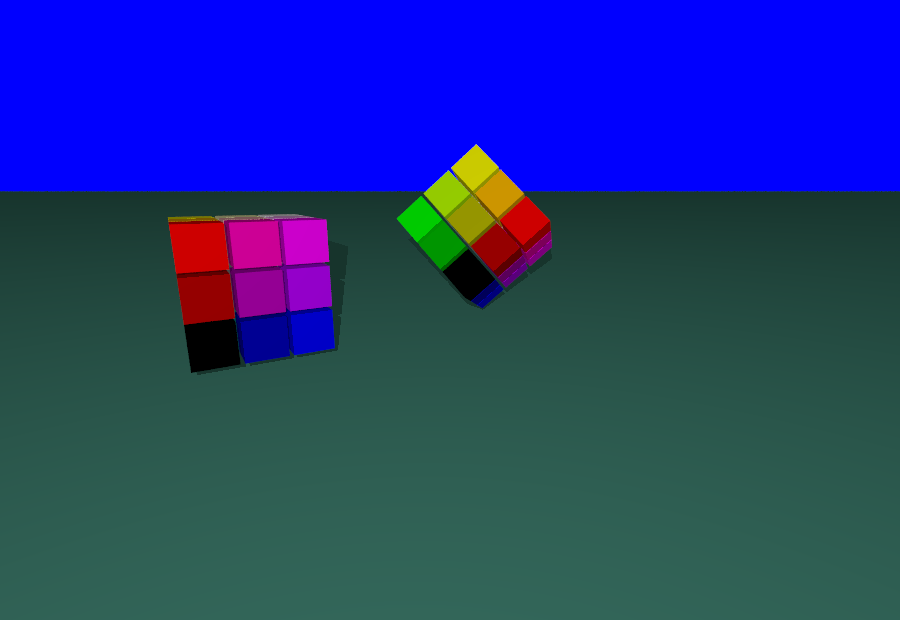# Objects and positions

In this note, we see how the basics to put an object at a precise location and illustrate the basics with some sort of Rubik’s cube.# a plane with normal the vector Z=vector(0,0,1)
ground=plane(Z,origin) .colored('Gray' )
basket=point(0,0,0)
cub=Cube(.91,.91,.91)
for a in range(3):
for b in range(3):
for c in range(3):
d=cub.copy()
d.color=" rgb <"+",".join([str(c),str(b),str(a)])+">"
d.move_at(point(a,b,c+0.5))
d.glued_on(basket)
# we copy the basket. The elements glued on it are also copied recursivly
basket2=copy.deepcopy(basket)
basket2.rotate(Segment(origin,origin+X+Y+Z),3.14/3)
basket2.translate(6*X+Z).glued_on(ground)
camera=Camera()
camera.file="rubik.pov"
camera.location=origin-10*Y+4*Z
camera.filmAllActors=False
camera.actors=[ground,basket] # what is seen by the camera wiht the children
camera.lookAt=.5*(basket2+basket)
camera.zoom(.2512)
camera.shoot # takes the photo, ie. creates the povray file, and stores it in camera.file
camera.show # show the photo, ie calls povray.


## During the creation

The basic way to put an object at a precise location is to do this at the time of creation. For instance,

Cube(point(a,b,c),point(x,y,z))


creates a cube with opposite corners (a,b,c) and (x,y,z).

## Moving an object already created

Often, it is not possible to place the object at the creation time. And often, the code is more clear when we separate the creation of the object and its positioning. For instance for the cube, we can declare the size of the cube, and move it afterwards.

c=Cube(2,3,4)
c.move_at(0,1,1)


This creates a cube of size(2,3,4) and puts its center at (0,1,1). The method move_at that we used for the cube may be used may be used for any bounded object : bounded objects carry a bounding box with them and the methods move_at puts the center of the bounding box at a precise location.

When the object c is not bounded, for instance a plane, or an infinite cylinder, it is still possible to move it using the methods translate and rotate whose signatures are as follows.

c.translate(x,y,z)
axis=Segment(point1,point2)
c.rotate(axis,angle)


When none of the above method works, it is still possible to use a general map M. For instance:

M=Map.linear(X,X+Y,Z)
c.move(M)


transforms the cube C by the linear map M sending the canonical base (X,Y,Z) to (X,X+Y,Z). A shortcut for c.move(Map.linear(a*X,b*Y,c*Z)) is

c.scale(a,b,c)


Using linear maps and translation, we can describe any affine map. The syntax is

M=Map.affine(a,b,c,w)
c.move(M)


which is equivalent to

M=Map.linear(a,b,c)
N=Map.translation(w)
c.move(N*M)